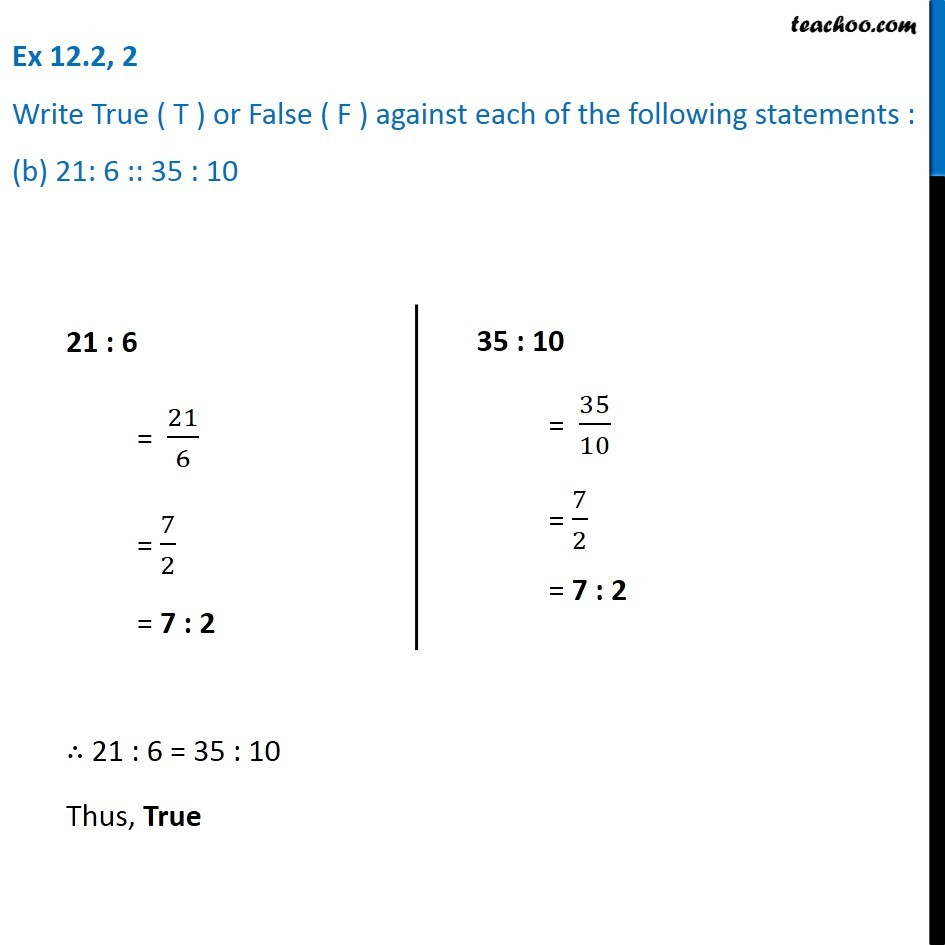Ex 12.2

Chapter 12 Class 6 Ratio and Proportion
Serial order wiseLearn in your speed, with individual attention - Teachoo Maths 1-on-1 Class

### Transcript

Ex 12.2, 2 Write True ( T ) or False ( F ) against each of the following statements : (b) 21: 6 :: 35 : 1021 : 6 = 21/6 = 7/2 = 7 : 2 35 : 10 = 35/10 = 7/2 = 7 : 2∴ 21 : 6 = 35 : 10 Thus, True